# Material Balance for Gas Reservoir

For gas reservoirs, the material balance concept can be applied to determine gas in place and expected gas reservoir reserve.

Gas Production = Expansion of Free Gas In Reservoir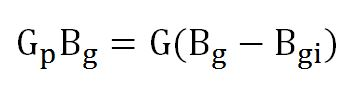Assumption:

• Dry gas reservoir
• No external energy support like water drive.

Where;

Gp = gas production (std cu-ft)

Bg = gas formation volume factor (res cu-ft/std cu-ft)

G = gas in place (std cu-ft)

Bgi = initial formation volume factor (res cu-ft/std cu-ft)

Gas formation volume factor (Bg)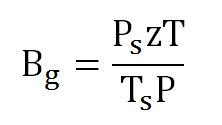Where;

Ps = pressure at standard condition (14.7 psia)

P = pressure at a certain condition, R

z = compressibility factor at a certain condition

T = temperature at a certain condition, R

T= standard temperature, 520 R (60F)

With Bg, the first equation can be written like this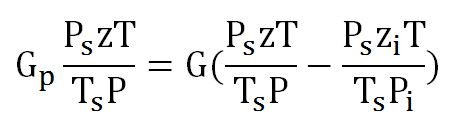Rearrange the equation like this: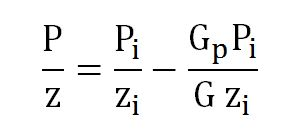The rearranged equation can be plotted between P/z and Gp (Figure 1).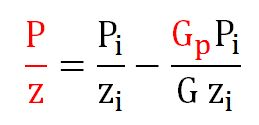Figure 1 – P/z plot

Figure 1 is called” P/z” plot and the interception at x-axis is gas in place (G).

Note: The P/z plot is valid for dry gas reservoir. If a reservoir has pressure support from an aquifer, the p/z plot will give an overestimated gas in place (Figure 2).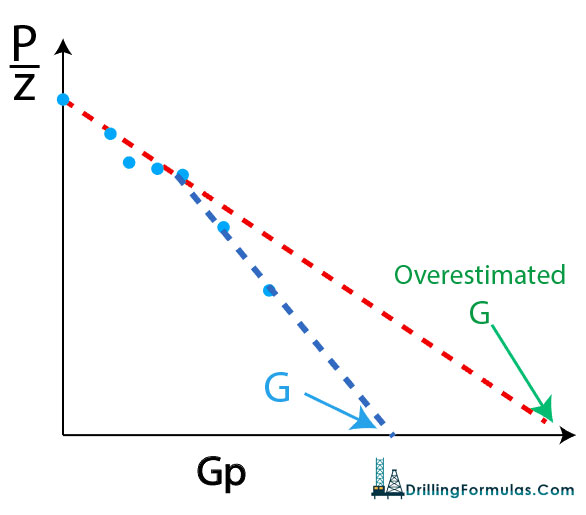Figure 2 – Over estimated gas in place

P/z plot is a good tool, but if you use the plot with few data points, it will give you a big error on gas in place (G) and reserves.

References

Abhijit Y. Dandekar, 2013. Petroleum Reservoir Rock and Fluid Properties, Second Edition. 2 Edition. CRC Press.

L.P. Dake, 1983. Fundamentals of Reservoir Engineering, Volume 8 (Developments in Petroleum Science). New impression Edition. Elsevier Science.

Tarek Ahmed PhD PE, 2011. Advanced Reservoir Management and Engineering, Second Edition. 2 Edition. Gulf Professional Publishing.

Share the joy
Tagged , , , . Bookmark the permalink.